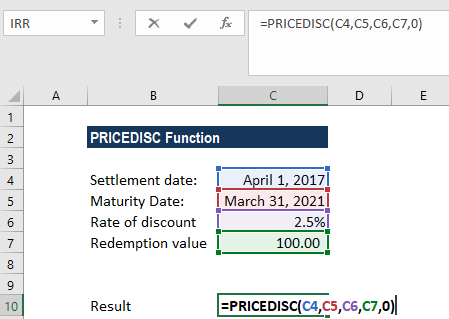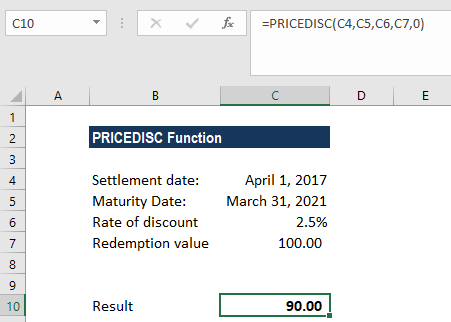# PRICEDISC Function

Calculates the price of a bond per \$100 face value of a discounted security

## What is the PRICEDISC Function?

The PRICEDISC function is categorized under Excel Financial functions. It will calculate the price of a bond per \$100 face value of a discounted security.

In financial analysis, PRICEDISC can be useful when we wish to borrow money by selling bonds instead of stocks. If we know the redemption value, interest rate, and the start and end dates, we can calculate the bond’s price using the function.

### Formula

=PRICEDISC(settlement, maturity, discount, redemption, [basis])

The PRICEDISC function uses the following arguments:

1. Settlement (required argument) – This is the security’s settlement date or the date the coupon is purchased. The security’s settlement date should be after the issue date.
2. Maturity (required argument) – This is the security’s maturity date or the date when the security expires.

To understand settlement and maturity, let’s take an example: a 30-year bond that was issued on January 1, 2017, and is purchased by a buyer six months later. The issue date would be January 1, 2017, the settlement date would be July 1, 2017, and the maturity date would be January 1, 2047, which is 30 years after the January 1, 2017 issue date.

1. Discount (required argument) – The discount rate of the security.
2. Redemption (required argument) – This is the redemption value of the security per \$100 face value.
3. Basis (optional argument) – This specifies the financial day count basis that is used by the security.

BasisDay Count basis
0 or omittedUS(NASD) 30/360
1Actual/actual
2Actual/360
3Actual/365
4European 30/360

### How to use the PRICEDISC Function in Excel?

As a worksheet function, PRICEDISC can be entered as part of a formula in a cell of a worksheet. To understand the uses of the function, let’s consider an example:

#### Example

Assume we wish to calculate the price per \$100 face value of a security that was discounted and purchased on April 1, 2017. The maturity date of security is March 31, 2021. The rate of discount is 2.5%. The redemption value is \$100. The US (NASD) 30/360 day count basis is used. We provide references to the cells in the formula.We get the result below:The above PRICEDISC function returns the value 90.00 (rounded off to 2 decimal points). The price of the security with the above terms would be \$90. In the above example:

1. As recommended by Microsoft Excel, we provided as input the settlement and maturity as references to cells containing dates.
2. The discount rate is provided in percentage form, 2.5%. However, the argument can instead be entered as the simple numerical value of 0.025.
3. If the [basis] argument is omitted, the function will use the default value 0 (denoting the US (NADS) 30/360 day count basis). Here, we provided it as 0.

### A few notes about the PRICEDISC Function:

1. #NUM! error – Occurs if either:
1. The settlement date is greater than or equal to maturity date;
2. When we provide invalid numbers for the arguments: the rate of discount, redemption or basis. That is, if we have provided a discount rate is less than or equal to zero, a redemption value is less than or equal to less than zero, or a basis that is a number other than 0,1,2,3,4.
2. #VALUE! error – Occurs if:
1. The given settlement or maturity arguments are invalid Excel dates.
2. Any of the given arguments are non-numeric.

Thanks for reading CFI’s guide to the Excel PRICEDISC function. By taking the time to learn and master these functions, you’ll significantly speed up your financial analysis. To learn more, check out these additional CFI resources:

• Excel Functions for Finance